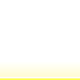Home » Blog » There is a real number between any two given real numbers.

# There is a real number between any two given real numbers.

Prove that ifwith, then there existssuch that.

Proof. (This is about the point in the blog at which I’m just going to use the basic properties ofwithout much comment. As usual, if something is unclear, leave a comment, and I’ll clarify.)Then,sinceand(sinceand using I.3.5, Exercise #4) and so their product is also greater than 0.
Then, by adding,So, letting, we havewithas requested1.Anonymous says:

Something went wrong with my comment. Here it is again:

There’s a problem with this argument.

As you mentioned, first you have to show that sup S = y. But, in order to show that, just by the definition of the supremum, you have to show that for any x<y, x is NOT an upper bound of S, which is exactly equivalent to there being a z in S (zx.

In other words, to show that sup S = y, you have to prove the theorem first! Which means that going the other way (proof of the theorem using sup S = y) would be wrong and circular reasoning.

•Anonymous says:

Ok, same problem again with my comment. It seems there’s something wrong with the website.

Basically, to show that sup S = y, where S is the set of all real numbers smaller than y, you have to prove this theorem first. So going the other way around would be wrong and circular reasoning.

(By the way I commented anonymously, but I’m not the same Anonymous as the previous Anonymous!)

2.alejandro says:

By absurd, if we suppose there is not a z for x<z<y then we imply that x=z and that is absurd since the letter says x<y.
If we form a set K with x as the Inf K and y as the Sup K, then there is a z in the real numbers for which Sup K – Inf K < z(theorem I.32).

•By definition, you can just say that for every

*** QuickLaTeX cannot compile formula:
z \in K

*** Error message:
Cannot connect to QuickLaTeX server: cURL error 60: Peer's Certificate issuer is not recognized.
Please make sure your server/PHP settings allow HTTP requests to external resources ("allow_url_fopen", etc.)
These links might help in finding solution:
http://wordpress.org/extend/plugins/core-control/
http://wordpress.org/support/topic/an-unexpected-http-error-occurred-during-the-api-request-on-wordpress-3?replies=37

; sup K

3.How do you develop the intuition to construct such a proof? How do you know where to start?

Thank you.

4.edward says:

at this point, how do we know that 2(y-x)>(y-x)?

•Anonymous says:

Because de teorem I.30 but he take n=2

•Anonymous says:

Thanks for the explanation. I was also thinking there is no theorem tells us that (y-x) + (y-x) = 2(y-x) at this point.

•Anonymous says:

Another possible explanation I thought of is the following:

As y-x>0, adding y-x to both sides and we get (y-x)+(y-x)>(y-x). Then we can form (y+y)-(x+x)>y-x. Using the distributive axiom, we get y(1+1)-x(1+1)>y-x. At this point we have the definition of 2 as 1+1. Then 2y-2x>y-x and thus 2(y-x)>y-x.

5.abomb says:

My version of anonymous’s solution which I like a lot more than the (x+y)/2 solution because we guarantee there exists a real instead of explicitly constructing one.

x, y ∈ ℝ such that x < y
S = { z y – h by T 1.32, that is z > x.
Hence there is a real z such that x < z < y.

•abomb says:

Yah, something went wrong too with my reply. It’s just basically anon’s solution but I was using fancy unicode, which I guess this site doesn’t have an encoding for. Oh well. Great site!!

6.Anonymous says:

Maybe an alternative solution:
Let S={s|sy – (y-x)=x
Hence we have x<z<y.

•Anonymous says:

Sorry but something went wrong as I posted the comment.
S is the set of all the real numbers that are smaller than y.
Apply theorem I.32 with h = y-x.
For some real number in S, say z, we have
z>y-(y-x)=x
Hence, x<z<y.

•Anonymous says:

the only thing left is you need to prove sup S = y before you use z>y-(y-x). Otherwise, only “THERE EXISTS z>Sup S – (y-x)” is true

•Anonymous says:

There’s a problem with this argument.

As you mentioned, first you have to show that sup S = y. But, in order to show that, just by the definition of the supremum, you have to show that for any x<y, x is NOT an upper bound of S, which is exactly equivalent to there being a z in S (zx.

In other words, to show that sup S = y, you have to prove the theorem first! Which means that going the other way (proof of the theorem using sup S = y) would be wrong and circular reasoning.

•Anonymous says:

Something went wrong with my comment too (weird…). Here is my comment whole again:

There’s a problem with this argument.

As you mentioned, first you have to show that sup S = y. But, in order to show that, just by the definition of the supremum, you have to show that for any x<y, x is NOT an upper bound of S, which is exactly equivalent to there being a z in S (zx.

In other words, to show that sup S = y, you have to prove the theorem first! Which means that going the other way (proof of the theorem using sup S = y) would be wrong and circular reasoning.